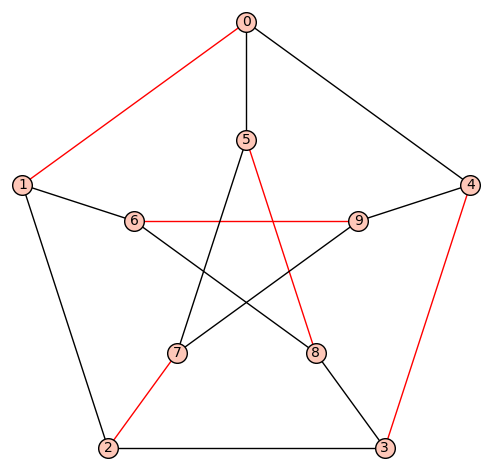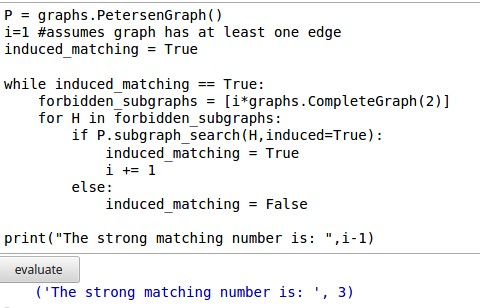Ask Your Question

# Find the induced matching

An induced matching in a graph G is a set of edges, no two of which meet a common node or are joined by an edge of G; that is, an induced matching is a matching which forms an induced subgraph.

Would you please tell me how I can find the max induced matching in a graph?

edit retag close merge delete

## 4 Answers

Sort by » oldest newest most voted

Here is a suggestion using mixed integer linear programming, see this tutorial for explicit Sage implementation:

• variables: to each edge $e$ of the graph $G$, we associate a boolean variables $m_e$ (telling whether $e$ belongs to the matching we are looking for)
• constraints:
• for each vertex $v$ of $G$, we want $\sum_{e \in N(v)} m_e \leq 1$ where $N(v)$ denotes the set of edges that are adjacent to the vertex $v$
• for each edge $e$ of $G$, we want $\sum_{f \in N(e)} m_f \leq 1$ where $N(e)$ denotes the set of edges that are adjacent to the edge $e$
• objective: we want to maximize the sum $\sum_{e\in G} m_e$

If you have any problem in writing this down into Sage code, do not hesitate to ask for details.

more

## Comments

@tmonteil, Thanks, I think it works, but I do not know how to write the code

Have you tried the alternative definition of taking an independent set in the square of the line graph ?

def square(G):
H = copy(G)
for u in G:
H.add_edges([(u, v) for v in G.vertex_boundary(G.neighbors(u, closed=True))])
return H

def induced_matching(G):
L = G.line_graph(labels=False)
return square(L).independent_set()


This gives

sage: G = graphs.PetersenGraph()
sage: induced_matching(G)
[(1, 6), (3, 4), (5, 7)]


EDIT: now method square returns H and not G... EDIT2: now method square is correct.

more

## Comments

Note that this is the same result as the one I obtain with dancing links which is apparently not an induced matching.

Method square was returning G instead of H... I fixed that and the result makes more sense now.

The strong matching number of the Petersen graph is 3. It looks like you are getting 2. How is your answer [(7,9)] supposed to be interpreted?

One solution is to use Knuth's dancing links algorithm which is implemented in Sage. Assuming the vertices of the graph are integers in the range(number_of_vertices), the method below will work:

from sage.combinat.matrices.dancing_links import dlx_solver
def find_matching(G):
rows = [[a,b] for (a,b,_) in G.edges()]
dlx = dlx_solver(rows)
solution = dlx.one_solution(ncpus=1)
if solution:
return [rows[a] for a in solution]
else:
return None


Then, on the Petersen graph, it gives:

sage: G = graphs.PetersenGraph()
sage: matching = find_matching(G)
sage: matching
[[2, 7], [0, 1], [5, 8], [3, 4], [6, 9]]
sage: G.plot(edge_colors={'red':matching})Another solution is to reduce the problem to SAT and use Sage SAT solvers or you may also review the ticket I wrote recently about the reduction of dancing links instance to SAT instance.

more

## Comments

@Sébastien you are showing a matching, not an induced matching, Indeed, since (0,1) and (6,9) are selected, (1,6) should be selected as well in the induced subgraph.

Oups! I needed to spend more time to understand the definition properly. Then, since we can not expect a perfect matching, it is more difficult to write as a tiling problem. I am now convinced the MILP is the way to go.

I'm unaware of anything built in to find the strong matching number. However, it's easy enough to put some code together. Sage is able to look for subraphs with subgraph_search. You can insist that the subgraph is induced as well. The Petersen graph has ten vertices so the strong matching number could be at most 5. The for loop has i run from 1 to 5, the forbidden subgraphs are set to i*graphs.CompleteGraph(2) and then Sage looks for that subgraph.

P = graphs.PetersenGraph()
i=1 #assumes graph has at least one edge
induced_matching = True

while induced_matching == True:
forbidden_subgraphs = [i*graphs.CompleteGraph(2)]
for H in forbidden_subgraphs:
if P.subgraph_search(H,induced=True):
induced_matching = True
i += 1
else:
induced_matching = False

print("The strong matching number is: ",i-1)


The result in a Sage Notebook is shown below.more

## Comments

@dazedANDconfused note that the agorthm used is brute force:

sage: from sage.graphs.generic_graph_pyx import SubgraphSearch
sage: SubgraphSearch??


Hence, it will likely not be able to handle medium-sized graphs.

The wikipedia page for induced matching that we were referred to says "...even finding a small induced matching of a given size k is unlikely to have an algorithm significantly faster than the brute force search approach of trying all k-tuples of edges.". I've used the Python3 syntax but you can see it doesn't print as you'd expect. I'm using the version of sage in my Linux versions repository. Python 2.7x print avoids that.

@dazedANDconfused -- regarding syntax, the following works in py2 and py3.

print("The strong matching number is {}".format(i - 1)")


## Your Answer

Please start posting anonymously - your entry will be published after you log in or create a new account.

Add Answer

## Stats

Asked: 2020-04-13 14:28:34 +0200

Seen: 202 times

Last updated: Apr 19 '20